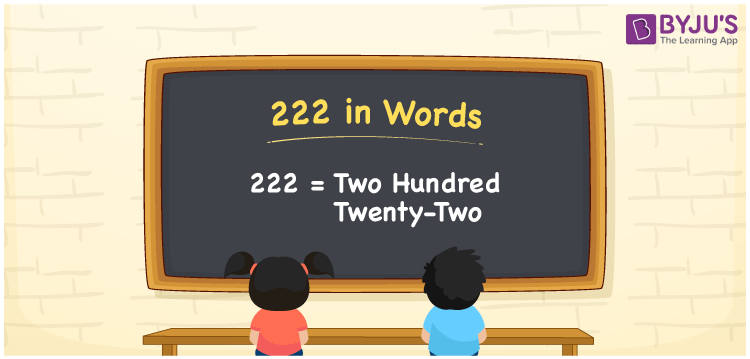# 222 in words

222 in words is written as Two Hundred and Twenty Two. 222 represents the count or value. The article on Counting Numbers can give you an idea about count or counting. The number 222 is a 3 digit number that is used in expressions related to money, days, distance, length, weight and so on. Let us consider an example for 222. “His lucky number is Two Hundred and Twenty Two.”

 222 in words Two Hundred and Twenty Two Two Hundred and Twenty Two in Numbers 222

## 222 in English Words## How to Write 222 in Words?

We can convert 222 to words using a place value chart. The number 222 has 3 digits, so let’s make a chart that shows the place value up to 3 digits.

 Hundreds Tens Ones 2 2 2

Thus, we can write the expanded form as:

2 × Hundred + 2 × Ten + 2 × One

= 2 × 100 + 2 × 10 + 2 × 1

= 222

= Two Hundred and Twenty Two.

222 is the natural number that is succeeded by 221 and preceded by 223.

222 in words – Two Hundred and Twenty Two.

Is 222 an odd number? – No.

Is 222 an even number? – Yes.

Is 222 a perfect square number? – No.

Is 222 a perfect cube number? – No.

Is 222 a prime number? – No.

Is 222 a composite number? – Yes.

## Solved Example

1. Write the number 222 in expanded form

Solution: 2 × 100 + 2 × 10 + 2 × 1

We can write 222 = 200 + 20 + 2

= 2 × 100 + 2 × 10 + 2 × 1.

## Frequently Asked Questions on 222 in words

Q1

### How to write the number 222 in words?

222 in words is written as Two Hundred and Twenty Two.
Q2

### Is 222 divisible by 3?

No. 222 is not divisible by 3.
Q3

### Is 222 a prime number?

No. 222 is not a prime number.